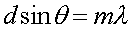Diffraction gratings

Diffraction gratings are glass plates with many parallel lines etched into the surface. Any two adjacent lines can be considered as a two slit setup, and the forumla,applies to find the angles where a maximum occurs in the interference pattern. The difference is that a much sharper image results. Consider an angle, just off the maximum, such that the path difference between the two paths is just one degree off the 360 degrees needed for a maximum. If one then considers the contribution from a slit 180 etchings away, that contribution will exactly cancel the first slit, and the intensity falls all the way to zero. Since the resulting lines are so sharp, a diffraction grating can be used to precisely measure wavelengths.

Examples     Interference and diffraction's index